## Exponential Distribution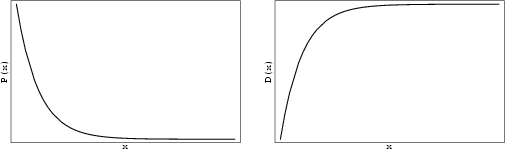Given a Poisson Distribution with rate of change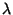, the distribution of waiting times between successive changes (with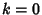) is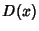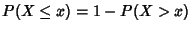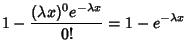(1)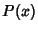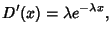(2)

which is normalized since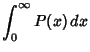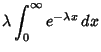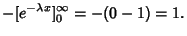(3)

This is the only Memoryless Random Distribution. Define the Mean waiting time between successive changes as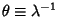. Then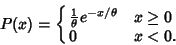(4)

The Moment-Generating Function is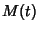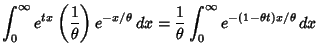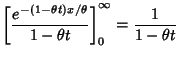(5)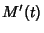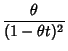(6)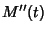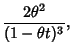(7)

so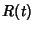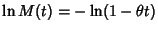(8)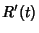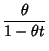(9)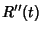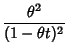(10)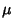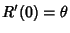(11)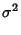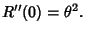(12)

The Skewness and Kurtosis are given by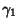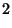(13)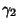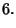(14)

The Mean and Variance can also be computed directly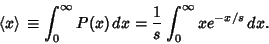(15)

Use the integral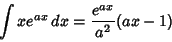(16)

to obtain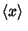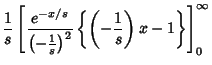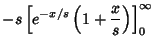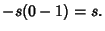(17)

Now, to find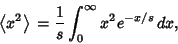(18)

use the integral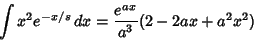(19)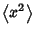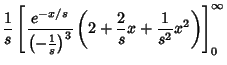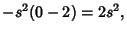(20)

giving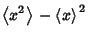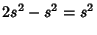(21)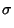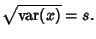(22)

If a generalized exponential probability function is defined by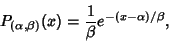(23)

then the Characteristic Function is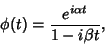(24)

and the Mean, Variance, Skewness, and Kurtosis are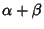(25)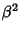(26)(27)(28)

References

Balakrishnan, N. and Basu, A. P. The Exponential Distribution: Theory, Methods, and Applications. New York: Gordon and Breach, 1996.

Beyer, W. H. CRC Standard Mathematical Tables, 28th ed. Boca Raton, FL: CRC Press, pp. 534-535, 1987.

Spiegel, M. R. Theory and Problems of Probability and Statistics. New York: McGraw-Hill, p. 119, 1992.